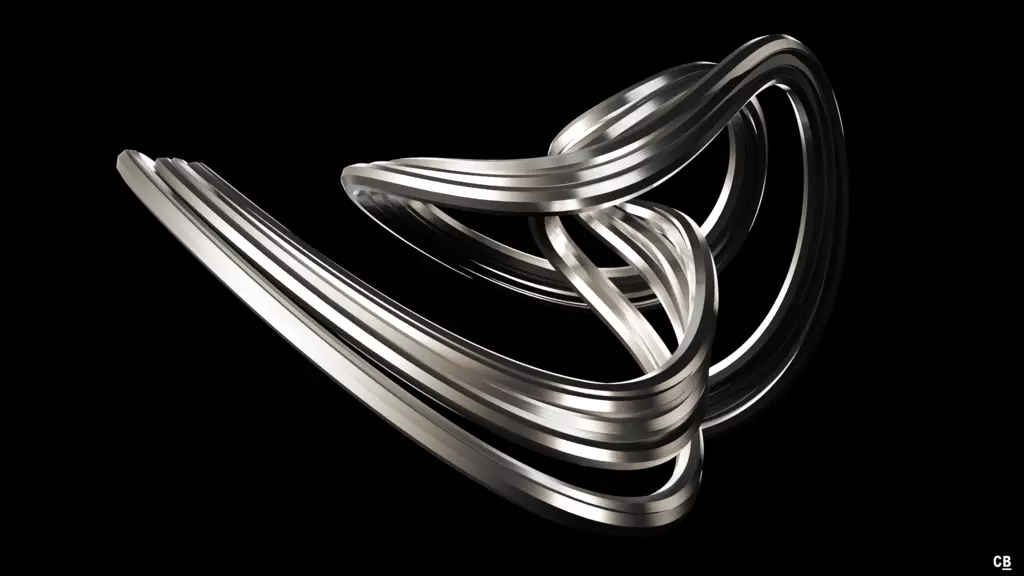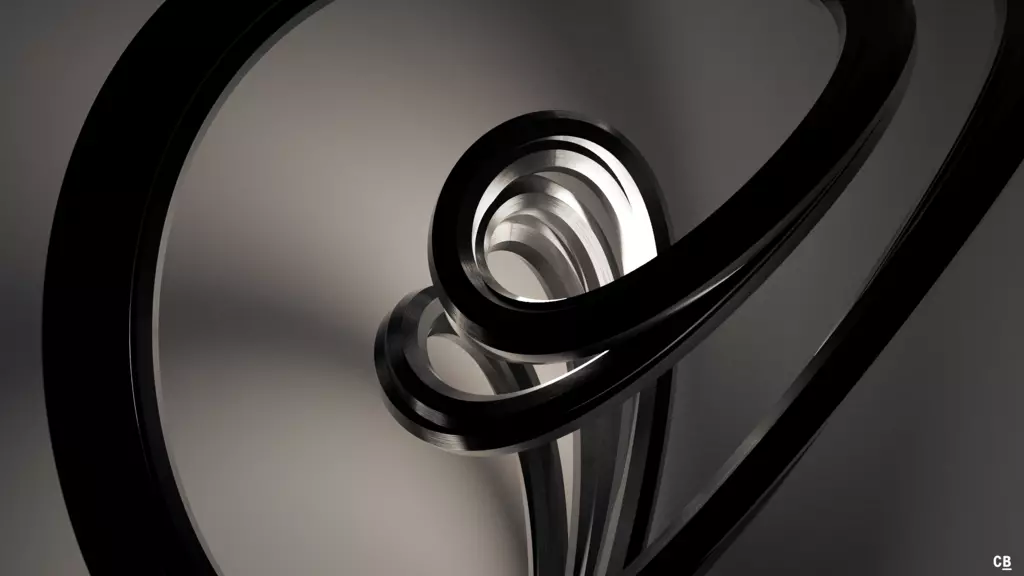# Sprott-G Attractor

This is case G of the 19 most simple nonlinear dynamical systems found by J.C. Sprott. Find more details on them and the first case A here.

## Renders

Differential system:

$\dot{x} = \alpha\, x + z$ $\dot{y} = x\, z - y$ $\dot{z} = -x + y$

Constants:

$\alpha = 0.4$## Stereographic Animation

You can now get a print of the Sprott attractors in my shop! Read more about it here.

🕸 💍